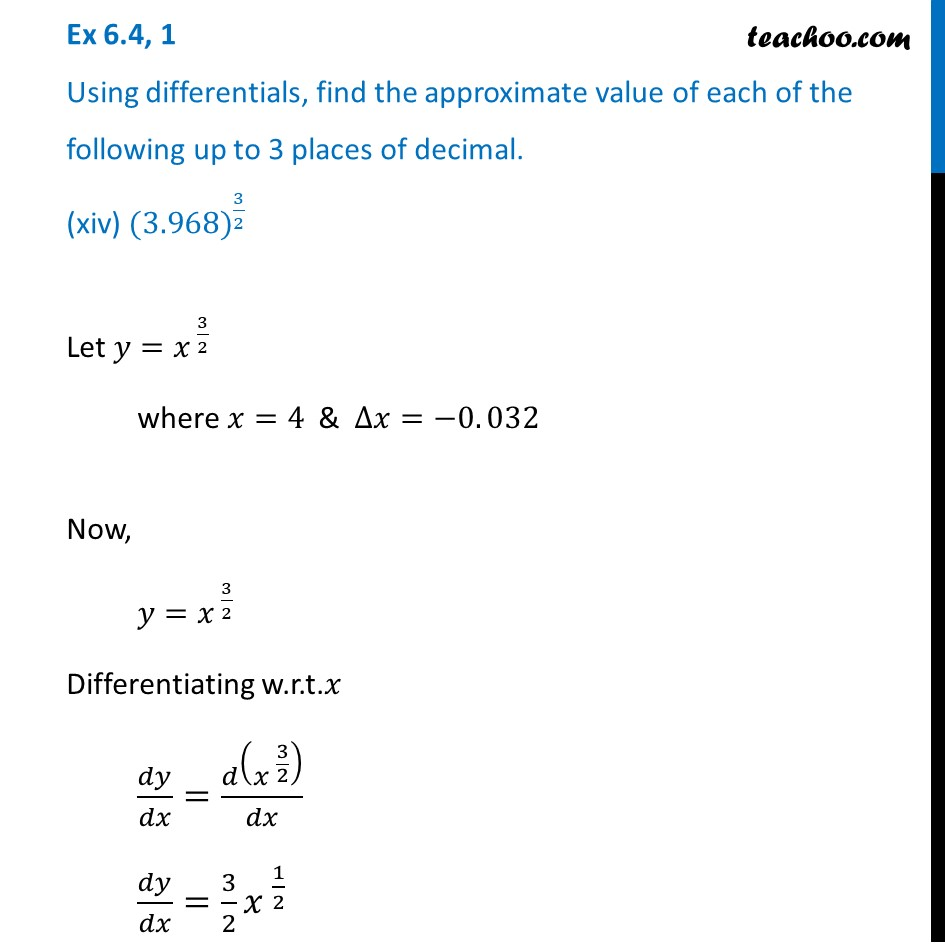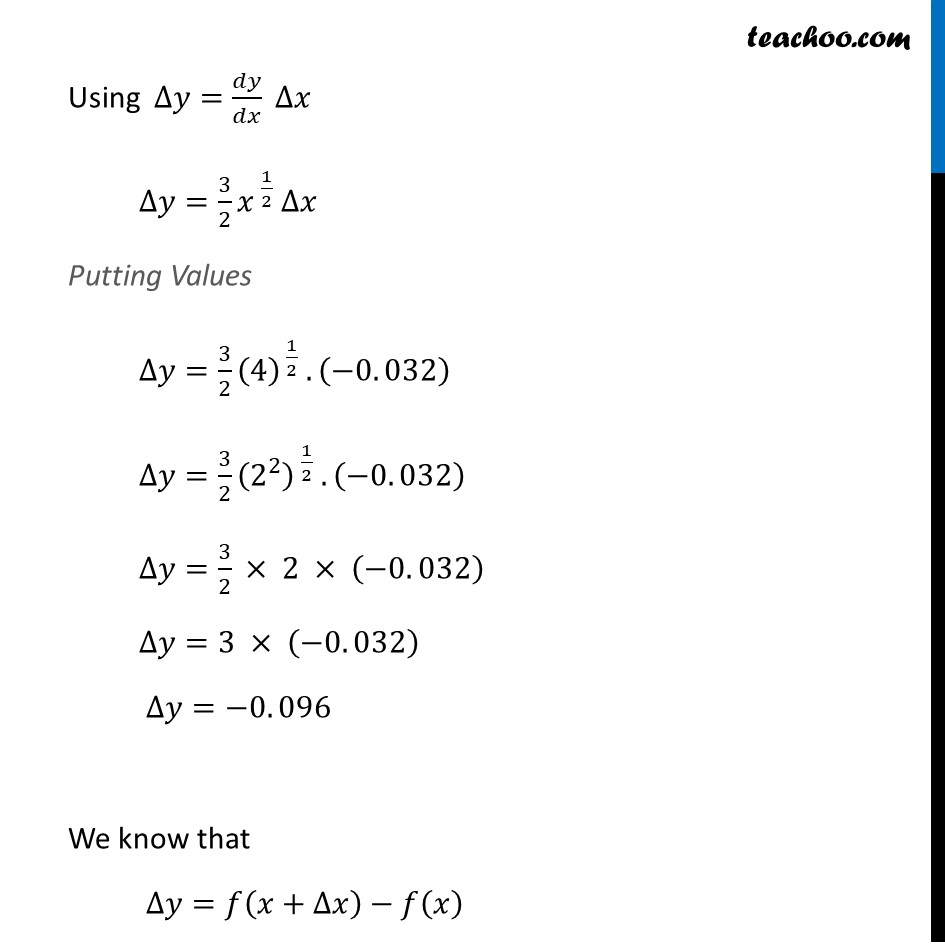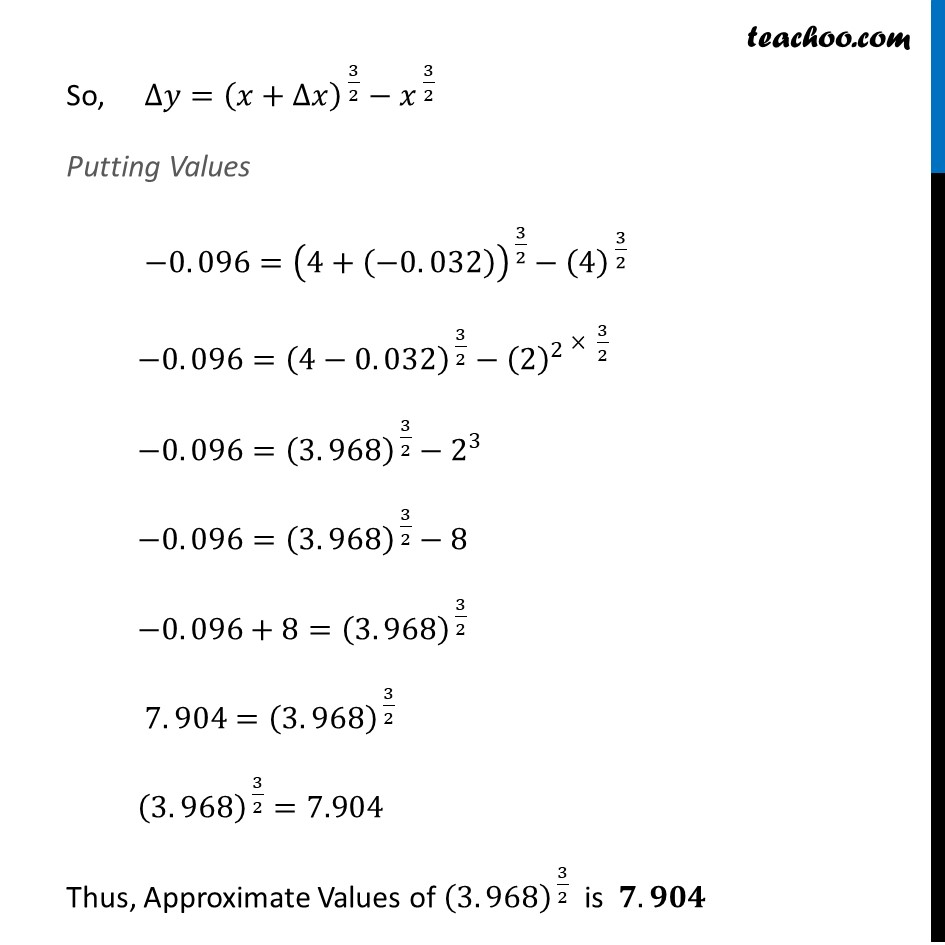Approximations (using Differentiation)

Chapter 6 Class 12 Application of Derivatives
Serial order wiseLearn in your speed, with individual attention - Teachoo Maths 1-on-1 Class

### Transcript

Question 1 Using differentials, find the approximate value of each of the following up to 3 places of decimal. (xiv) 〖(3.968)〗^(3/2)Let 𝑦=𝑥^( 3/2) where 𝑥=4 & ∆𝑥=−0. 032 Now, 𝑦=𝑥^( 3/2) Differentiating w.r.t.𝑥 𝑑𝑦/𝑑𝑥=𝑑(𝑥^( 3/2) )/𝑑𝑥 𝑑𝑦/𝑑𝑥=3/2 〖𝑥 〗^(1/2) Using ∆𝑦=𝑑𝑦/𝑑𝑥 ∆𝑥 ∆𝑦=3/2 𝑥^( 1/2) ∆𝑥 Putting Values ∆𝑦=3/2 (4)^( 1/2) . (−0. 032) ∆𝑦=3/2 (2^2 )^( 1/2) . (−0. 032) ∆𝑦=3/2 × 2 × (−0. 032) ∆𝑦=3 × (−0. 032) ∆𝑦=−0. 096 We know that ∆𝑦=𝑓(𝑥+∆𝑥)−𝑓(𝑥) So, ∆𝑦=(𝑥+∆𝑥)^( 3/2)−𝑥^( 3/2) Putting Values −0. 096=(4+(−0. 032))^( 3/2)−(4)^( 3/2) −0. 096=(4−0. 032)^( 3/2)−〖(2)^2〗^( × 3/2) −0. 096=(3. 968)^( 3/2)−2^3 −0. 096=(3. 968)^( 3/2)−8 −0. 096+8=(3. 968)^( 3/2) 7. 904=(3. 968)^( 3/2) (3. 968)^( 3/2)=7.904 Thus, Approximate Values of (3. 968)^( 3/2) is 𝟕. 𝟗𝟎𝟒